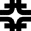[HOME]Diffractive Dijets with a Leading Antiproton in pbar-p Collisions at sqrt(s)=1800 GeVCDF Collaboration

Phys. Rev. Lett. 84, 5043

Abstract
We report results from a study of events with a leading antiproton of beam momentum fraction 0.905< xF <0.965 and 4-momentum transfer squared |t|<3 GeV^2 produced in pbar-p collisions at sqrt(s)=1800 GeV at the Fermilab Tevatron collider. Approximately 2% of the events contain two jets of transverse energy ETjet >7 GeV. Using the dijet events, we evaluate the diffractive structure function of the antiproton and compare it with expectations based on results obtained in deep inelastic scattering experiments at the DESY ep collider HERA.

Figures
 Fig. 1 Distributions versus xi and t: (a) Roman pot acceptance; (b) inclusive diffractive event sample; (c) ratio of dijet to inclusive diffractive events versus xi and (d) versus t. Fig. 2 Comparison of diffractive to non-diffractive dijet (a) mean ET and (b) mean eta distributions. Fig. 3 Ratio of diffractive to non-diffractive dijet event rates as a function of x (momentum fraction of parton in pbar). The solid lines are fits to the form R(x)=Ro(x/0.0065)-r for beta<0.5. (The data points of this figure are here. The data points of the R(x) distribution for 0.035< xi <0.095 are here.) Fig. 4 Data beta distribution (points) compared with expectations from the parton densities of the proton extracted from diffractive deep inelastic scattering by the H1 Collaboration. The straight line is a fit to the data of the form beta-n. The lower (upper) boundary of the filled band represents the data distribution obtained by using only the two leading jets (up to four jets of ET>5 GeV) in evaluating beta. The dashed (dotted) lines are expectations from the H1 fit 2 (fit 3). All the distributions in this figure are normalized per unit xi. The systematic uncertainty in the normalization of the data is +/- 25%. (The data points of this figure are here.)

References

1. H1 Collaboration, T. Ahmed et al., Phys. Lett. B 348, 681 (1995) [H1] ; C. Adloff et al., Z. Phys. C 76, 613 (1997). [Z.Phys.C |H1]
2. ZEUS Collaboration, M. Derrick et al., Z. Phys. C 68, 569 (1995) [ZEUS] ;Phys. Lett. B 356, 129 (1995) [ZEUS] ; Eur. Phys. J. C 6, 43 (1999). [Eur.Phys.J.C |ZEUS]
3. UA8 Collaboration, A. Brandt et al., Phys. Lett. B 297, 417 (1992). [CERN]
4. CDF Collaboration, F. Abe et al., Phys. Rev. Lett. 78, 2698 (1997) [PRL |CDF]; 79, 2636 (1997) [PRL |CDF]; T. Affolder et al., Phys. Rev. Lett. 84 232 (2000). [PRL |CDF]
5. We use rapidity and pseudorapidity, eta, interchangeably; eta = -ln(tan(theta/2)), where theta is the polar angle of a particle with respect to the proton beam direction. The azimuthal angle is denoted by phi, and the transverse energy of a jet, ETjet, is defined as Ejetsin(theta).
6. See, for example, P.D.B. Collins, An Introduction to Regge Theory and High Energy Physics, Cambridge University Press, Cambridge (1977).
7. K. Goulianos, in Proceedings of ``Vth International Workshop on Deep Inelastic Scattering and QCD", Chicago, USA, 1997, edited by J. Repond and D. Krakauer (AIP Conf. Proc. 407, 1997) pp. 527-532.
8. L. Alvero, J. C. Collins, J. Terron and J. Whitmore, Phys. Rev. D 59, 074022 (1999). [PRD| |LANL]
9. R.J.M. Covolan and M.S. Soares, Phys. Rev. D 60, 054005 (1999); erratum in D 61, 019901 (2000). [PRD |LANL]
10. F. Abe et al., Nucl. Instrum. Methods A 271, 387 (1988). [CDF]
11. F. Abe et al., Phys. Rev. D 50, 5518 (1994) [PRD]; D 50, 5535 (1994) [PRD]; D 50, 5550 (1994) [PRD].
12. F. Abe et al., Phys. Rev. D 45, 1448 (1992). [PRD]
13. M. Glück, E. Reya and A. Vogt, Eur. Phys. J. C 5, 461 (1998). [Eur.Phys.J.C |LANL]
14. J.F. Owens, Phys. Rev. D 30, 943 (1984). [PRD]
15. Private communications with Armen Bunyatyan, Hannes Jung and Chistophe Royon.
16. K. Goulianos, Phys. Lett. B 358, 379 (1995); B 363, 268 (1995).